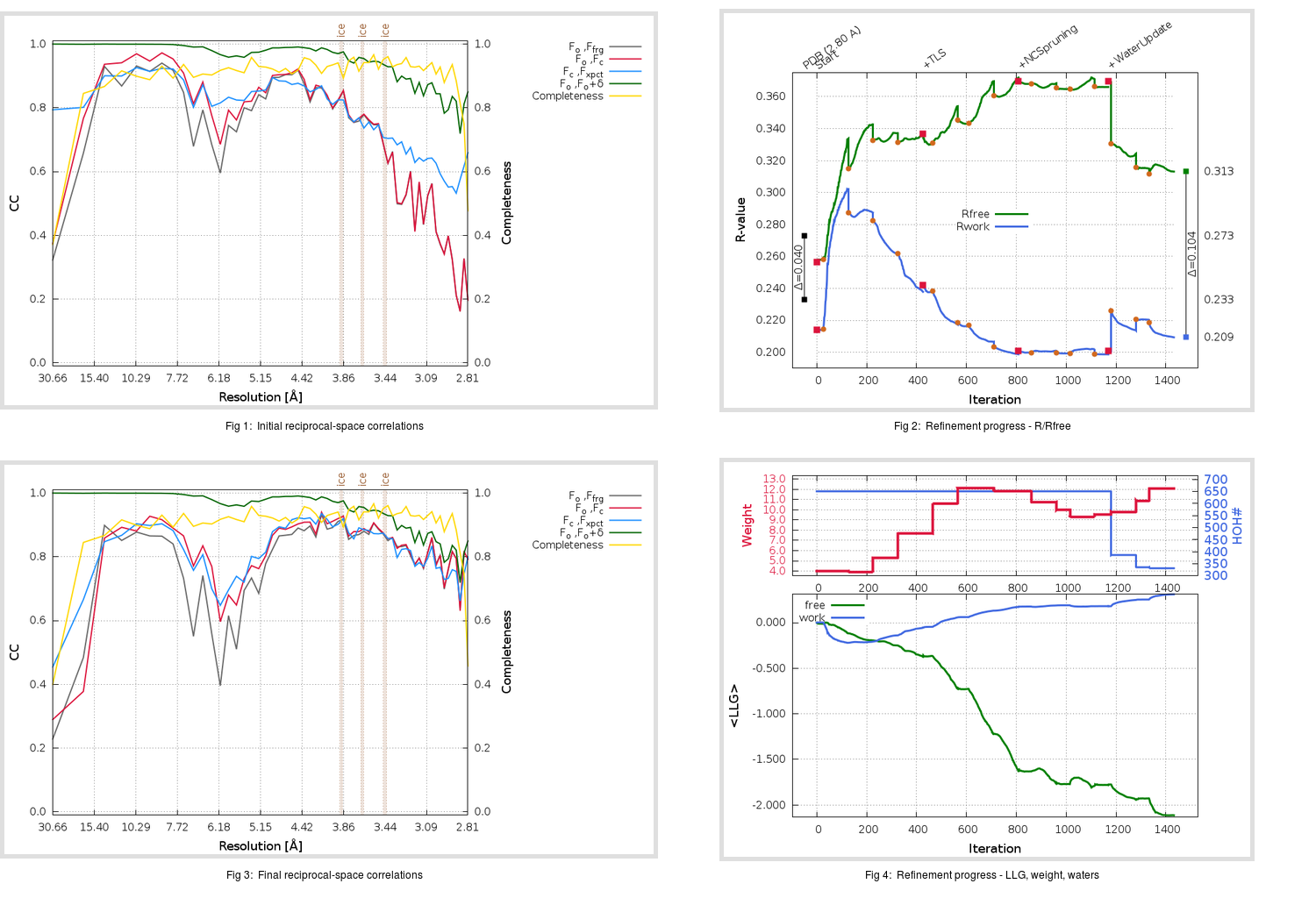Content:

```    Diffraction limits & principal axes of ellipsoid fitted to diffraction cut-off surface:
3.395         0.9534   0.0000   0.3018       a* + 0.002 c*
2.636         0.0000   1.0000   0.0000       b*
2.584        -0.3018   0.0000   0.9534      -0.463 a* + 0.886 c*
```

## Deposited

` `
 Date deposited Date data collection Resolution R, Rfree 20040729 2.80 0.2330 0.2730

Molprobity (CCP4 7.0 version) summary:

```Ramachandran outliers =  16.51 %
favored =  61.86 %
Rotamer outliers      =  15.66 %
C-beta deviations     =     1
Clashscore            = 151.03
RMS(bonds)            =   0.0142
RMS(angles)           =   2.00
MolProbity score      =   4.45
Resolution            =   2.80
R-work                =   0.2330
R-free                =   0.2730
```

```Number of waters      =   651

<B> (all atoms) =   43.46 ( sd =   18.90 ) for       4131 non-hydrogen atoms
<B>   (protein) =   42.07 ( sd =   18.51 ) for       3480 non-hydrogen atoms
<B>     (water) =   50.88 ( sd =   19.22 ) for        651 non-hydrogen atoms
<B>    (others) =    0.00 ( sd =    0.00 ) for          0 non-hydrogen atoms

B min/max       (all non-hydrogen atoms) =    1.20 /  120.20
B min/max   (protein non-hydrogen atoms) =    1.20 /   97.32
B min/max     (water non-hydrogen atoms) =    7.93 /  120.20
B min/max     (other non-hydrogen atoms) =    0.00 /    0.00
```

## BUSTER (re-)refinement

` `

Molprobity (CCP4 7.0 version) summary:

```Ramachandran outliers =   0.47 %
favored =  97.91 %
Rotamer outliers      =  12.88 %
C-beta deviations     =     0
Clashscore            =  11.28
RMS(bonds)            =   0.0119
RMS(angles)           =   1.45
MolProbity score      =   2.43
Resolution            =   2.80
R-work                =   0.2094
R-free                =   0.3131
```

```Number of waters      =   331

<B> (all atoms) =   65.06 ( sd =   13.63 ) for       3811 non-hydrogen atoms
<B>   (protein) =   66.61 ( sd =   12.39 ) for       3480 non-hydrogen atoms
<B>     (water) =   48.79 ( sd =   15.33 ) for        331 non-hydrogen atoms
<B>    (others) =    0.00 ( sd =    0.00 ) for          0 non-hydrogen atoms

B min/max       (all non-hydrogen atoms) =   14.11 /  110.89
B min/max   (protein non-hydrogen atoms) =   42.49 /  110.89
B min/max     (water non-hydrogen atoms) =   14.11 /  105.37
B min/max     (other non-hydrogen atoms) =    0.00 /    0.00
```

Refinement progression:Results:

` `
 File Remark 1WNC_aB_refine.01_04_refine.pdb.gz exact refinement commands are in header 1WNC_aB_refine.01_04_refine.mtz.gz including original deposited data and several re-refinement map coefficients 1WNC_aB_refine.01_04_BUSTER_model.cif.gz including any non-standard compound restraints 1WNC_aB_refine.01_04_BUSTER_refln.cif.gz